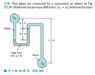# Manometer problem solving.

• aaronfue

## Homework Statement

Two pipes are connected by a manometer shown in the figure.

## Homework Equations

FR = $\gamma$*hc*A

yR = yc + $\frac{I_xc}{y_c*A}$

## The Attempt at a Solution

PB - PA = $\gamma$water*1.1m - $\gamma$Fluid*0.6m + $\gamma$water*0.8m = 3.33 kPa

I checked my answer and it was all okay except the answer was supposed to be -3.33 kPa. Can someone brief me on why that is?

#### Attachments

•fluids 2-30.JPG
13.2 KB · Views: 955
The problem asked for PA-PB, and you calculated PB-PA.

•1 person